### Alternating Current Class 12th Physics Part I CBSE Solution

##### Question 1.An LC circuit contains a 20 mH inductor and a 50 μF capacitor with an initial charge of 10 mC. The resistance of the circuit is negligible. Let the instant the circuit is closed be t = 0.(a) What is the total energy stored initially? Is it conserved during LC oscillations?(b) What is the natural frequency of the circuit?(c) At what time is the energy stored(i) completely electrical (i.e., stored in the capacitor)? (ii) completely magnetic (i.e., stored in the inductor)?(d) At what times is the total energy shared equally between the inductor and the capacitor?(e) If a resistor is inserted in the circuit, how much energy is eventually dissipated as heat?Answer:Inductor L = 20 mHIn henry L = 20 × 10-3Hcapacitor C = 50 μF = 50 × 10-6FCharge Q = 10 mC = 10 × 10-3C(a) Total energy stored in the capacitor can be calculated as follows:E = 1/2 Q2CSubstituting values in above formula, we getE =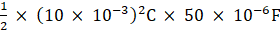On calculating, we getE = 1 JouleYes, the total energy will be conserved during LC oscillations because there is no resistor connected in the circuit.(b) Natural frequency of the circuit can be calculated using the following expression:V = 1/(2π√LC)V = 1/[2 × 3.14 × √ (20 × 10-3H × 50 × 10-6F)]On calculating, we getV = 159.24 HzNatural angular frequency is given by the following expression:ωr = 1/√ LCor ωr = 1/√ (20 × 10-3H × 50 × 10-6F)ωr = 103 rad/s(c) (i) Time period T = 1/vT = 1/159.24 HzOr T = 6.29 msTherefore, for time period T = 6.29 ms, the total charge on the capacitor can be calculated by the following relation:Q’ = Q cos 2π/TSince energy stored is electrical, therefore Q’ = QTherefore, energy stored is electrical at time t = 0, T/2, T, 3T/2(ii) Since when electrical energy is zero, the magnetic energy will be maximumTherefore, energy stored is electrical at time t = T/4, 3T/4, 5T/4(d) Q’ is equal to the charge on the capacitor when the total energy is shared between capacitor and inductorWhen the energy is shared between the capacitor and inductor then the total energy stored in the capacitor is given as half of the maximum energy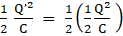⇒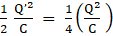⇒ Q’ = Q/√ 2………………(1)But as we know, Q’ = Q cos 2π/TSubstituting eqn (1) in above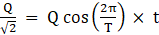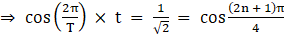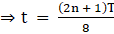Thus, the total energy is shared between capacitor and inductor at time t = T/8, 3T/8, 5T/8(e) If a resistor is inserted in the circuit, then total energy is eventually dissipated as heat.Question 2.A coil of inductance 0.50 H and resistance 100 W is connected to a 240 V, 50 Hz A.C. supply.(a) What is the maximum current in the coil?(b) What is the time lag between the voltage maximum and the current maximum?Answer:Given: Inductance = 0.50 HResistance R = 100 ΩVoltage V = 240 VFrequency = 50 HzThe diagram is: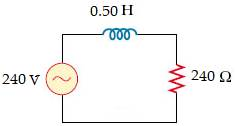(a) Peak voltage is given by the following formula:V0 = √2 VV0 = √2 × 240VOn calculating, we getV0 = 339.41 VAngular frequency is given by the formula ω = 2πvSubstituting value of v in above formulaω = 2 × π × 50(Hz) = 100 π rad/s.The maximum current in the coil can be calculated as follows: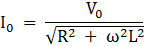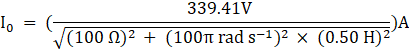On calculating, we getI0 = 1.82 A(b) The equation for voltage and current are as follows:V = V0 cos ωtI = I0 cos (ωt-Ф)At time t = 0, V = V0At t = Ф/ ω, I = I0Therefore, the time lag between maximum current and maximum voltage is Ф/ ω. Phase angle is given by the following relation:tan Ф = ωL/Rtan Ф = (2 π × 50(Hz) × 0.5(H))/100On calculating, we gettan Ф = 1.57or Ф = 57.50in rad Ф = 57.5π/180 radωt = 57.5π/180or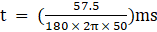On calculating, we gett = 3.2 msQuestion 3.Obtain the answers (a) to (b) in Exercise 13 if the circuit is connected to a high frequency supply (240 V, 10 kHz). Hence, explain the statement that at very high frequency, an inductor in a circuit nearly amounts to an open circuit. How does an inductor behave in a dc circuit after the steady state?Answer:Given: Inductor = 0.5 HzResistor R = 100 ΩVoltage V = 240 VFrequency v = 10 kHzIn Hz, the frequency v = 104 Hz(a) Angular frequency is given by the following relationω = 2πvω = 2π × 104 rad/sPeak voltage is given by the relation: V = √2 × VOn substituting the valuesV = 240√2VThe maximum current is given by the relation:Substituting the values we get⇒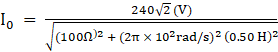On calculating, we get⇒ I0 = 1.1 × 10-2A(b) tan Ф = ωL/Rtan Ф =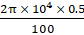on calculating, we getor Ф = = 89.820in rad, Ф = 89.82 π/180 radωt = 89.82π/180or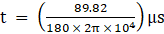On calculating, we gett = 25 μsSince I0 is very small, therefore at higher frequency the inductor is at open circuit.In case of a dc circuit, when steady state is obtained i.e. ω = 0. Therefore, the inductor behaves like a conducting object.Question 4.A 100 μF capacitor in series with a 40Ω resistance is connected to a 110 V, 60 Hz supply.(a) What is the maximum current in the circuit?(b) What is the time lag between the current maximum and the voltage maximum?Answer:Given: capacitor C = 100 μf = 100 × 10-6FResistance R = 40ΩVoltage V = 110 VFrequency v = 60 Hz(a) Angular frequency is given by the relation: ω = 2πvω = 2π × 60 rad/sThe impedance is given by the relation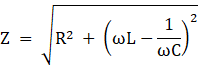Or Z2 =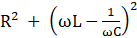Peak voltage is given by the relation V0 = V √2Or V0 = 110 √ 2 VThe maximum current is given by the following relation:I0 = V0/ZI0 =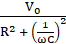I0 =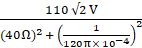On calculating, we getI0 = 3.24 A(b) Since in capacitor circuit, the voltage lags behind the current by a phase angle Ф. Therefore, the phase angle is given by the expressiontanФ =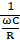tanФ = 1/ωCRsubstituting the values, we gettanФ = 1/(120π(rad/s) × 10-4(F) × 40 (Ω))On calculating, we gettanФ = 0.6635or Ф = 33.56°in radians, Ф = (33.56 π) /180Time lag is given by the relationt = Ф/ωt =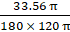on calculating, we gett = 1.55 × 10-3son converting in ms, we get time lag t = 1.55 ms.Question 5.Obtain the answers to (a) and (b) in Exercise 15 if the circuit is connected to a 110 V, 12 kHz supply? Hence, explain the statement that a capacitor is a conductor at very high frequencies. Compare this behaviour with that of a capacitor in a dc circuit after the steady state.Answer:Given: Capacitance C = 100 μFIn Farad, Capacitance C = 100 × 10-6FResistance = 40ΩVoltage V = 110 VFrequency = 12 kHzIn Hz, frequency v = 12 × 103HzAngular frequency ω = 2πv = 2 × π × 12 × 103(Hz)ω = 24 × 103 rad/sPeak voltage is calculated as follows:V0 = V/ √2V0 = 110√2VThe peak current is calculated as follows:I0 =I0 =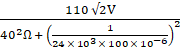On calculating, we getI0 = 3.9 ASince for RC circuit, the voltage lags behind the current by a phase angle of ФtanФ =tanФ = 1/ωCRsubstituting the values, we gettanФ = 1/(24π × 103(Hz) × 100 × 10-6(F) × 40 (Ω))On calculating, we gettanФ = 1/96πor Ф = 0.20in radians, Ф = (0.2 π) /180At high frequency, Ф approaches to zero and capacitor acts as a conductor.In d.c. circuit, ω is zero, and the steady state is attained and therefore, capacitor C tends to be an open circuit.Question 6.Keeping the source frequency equal to the resonating frequency of the series LCR circuit, if the three elements, L, C and R are arranged in parallel, show that the total current in the parallel LCR circuit is minimum at this frequency. Obtain the current rms value in each branch of the circuit for the elements and source specified in Exercise 11 for this frequency.Answer:Given: L = 5.0 Hcapacitor C = 80 × 10-6FResistance = 40 ΩVoltage = 230 VImpedance is expressed as: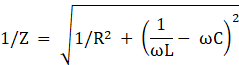At resonance condition,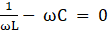ω = 1/ √LCsubstituting the values, we getω = 1/ √(5(H) × 80 × 10-6(F))On calculating, we getω = 50 rad/sSince, the magnitude of impedance is maximum at 50 rad/s due to which the total current is minimum.The root mean square current flowing through the inductor is given by the relation:IL = V/ωLSubstituting the values, we getIL = 230(V)/(50 (rad/s) × 5 (H))On calculating, we getIL = 0.92 ARoot mean square value of the current through capacitor is given by the following relation:Ic = ωCVIc = 50rad/s × 80 × 10-6F × 230V = 0.92 ARoot mean square value of the current through resistor is given by the following relation:IR = V/RIR = 230V/40ΩIR = 5.75AQuestion 7.A circuit containing a 80 mH inductor and a 60 μF capacitor in series is connected to a 230 V, 50 Hz supply. The resistance of the circuit is negligible.(a) Obtain the current amplitude and rms values.(b) Obtain the rms values of potential drops across each element.(c) What is the average power transferred to the inductor?(d) What is the average power transferred to the capacitor?(e) What is the total average power absorbed by the circuit? [‘Average’ implies ‘averaged over one cycle’.]Answer:Given: L = 80 mH = 80 × 10-3 Hcapacitor C = 60 Μf = 60 × 10-6 FVoltage V = 230 VFrequency v = 50Hz(a) Peak voltage is given by the formula:V0 = V/√ 2V0 = 230√2 V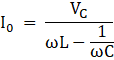Substituting the values we get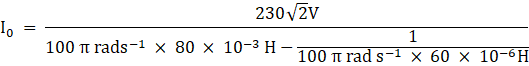On calculating, we getI0 = -11.63 A.The amplitude of maximum current is given by the following relation:I0 = 11.63 A.The root mean square value of the current I = I0/√ 2⇒ I = -11.63(A)/√ 2⇒ I = - 8.22 A(b) The potential difference across the inductor can be calculated as follows:VL = I × ω × L = 8.22(A) × 100 π(Hz) × 80 × 10-3 (F) = 206.61 VPotential difference across the capacitor is given by the formula as follows:Vc = I × 1/ωCSubstituting values we getVc = 8.22(A) × 1/ 100 π(Hz) × 60 × 10-6(F)On calculating, we getVc = 436.3 V(c) Since the actual voltage leads the current by π/2, so the average power consumed by the inductor is zero.(d) Since voltage lags the current by π/2, so the average power consumed by the capacitor is zero.(e) The total power over a complete cycle is zero.Question 8.Suppose the circuit in Exercise 18 has a resistance of 15Ω. Obtain the average power transferred to each element of the circuit, and the total power absorbed.Answer:Given: Average power transferred to the resistor = 788.44 WAverage power transferred to the capacitor = 0 WPower absorbed by the circuit = 788.44 WInductance L = 80 mHIn henry, Inductance L = 80 × 10-3HCapacitance C = 60 μF = 60 × 10-6FResistance R = 15 ΩVoltage V = 230 VFrequency v = 50 HzAngular frequency is calculated as follows:ω = 2πvω = 2 × 3.14 × 50(Hz) = 100π rad/sThe impedance can be calculated as follows: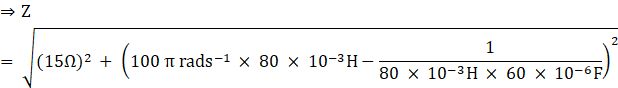On calculating, we getZ = 31.728 ΩCurrent can be calculated as follows: I = V/ZI = 230 (A)/31.728 (Ω) = 7.25 AThe average power transferred to resistor can be calculated as follows:Pr = I2RSubstituting values, we getPr = (7.25)2(A) × 15(Ω)Pr = 788.44 WThe average power transferred to capacitor is equal to the average power transferred to resistor = 0Total power absorbed = PR + Pc + PL = 788.4WQuestion 9.A series LCR circuit with L = 0.12 H, C = 480 nF, R = 23Ω is connected to a 230 V variable frequency supply.(a) What is the source frequency for which current amplitude is maximum. Obtain this maximum value.(b) What is the source frequency for which average power absorbed by the circuit is maximum. Obtain the value of this maximumpower.(c) For which frequencies of the source is the power transferred to the circuit half the power at resonant frequency? What is the current amplitude at these frequencies?(d) What is the Q-factor of the given circuit?Answer:(a) Given: L = 0.12 HC = 480 nFConverting into Farad, we get 480 × 10-9FR = 23ΩVoltage = 230 VPeak voltage can be calculated as follows:V0 = √2VSubstituting values we getV0 = 230√2V0 = 325.22 VCurrent flowing through the circuit is given by the following:I0 =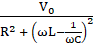At resonating frequency, ωR L - 1/ωRC = 0ωR = 1/√LC= 1/ √0.12 (H) × 480 × 10-9 (F) = 4166.67 rad/sResonant frequency can be calculated using the formula:vR = ωR/2πvR = 4166.67(Hz)/2 × 3.14vR = 663.48 HzMaximum current is calculated as follows:(I0)max = V0/R = 325.22(Hz)/23 = 14.14 A(b) The maximum power absorbed by the circuit can be calculated as follows:Pav = 1/2(I0)2maxRSubstituting the values, we getPav = 1/2 × (14.14)2(A) × 23 (Ω)Pav = 2299.33 WTherefore, the resonating frequency is 663.48 Hz.(c) The power transferred is equal to the half of the power at the resonating frequency.Frequency at which power is half = ωR �∆ωOr = 2π (vR �∆v)Where ∆ω = R/2LSubstituting the values we get∆ω = 23/2(Ω) × 0.12(H)∆ω = 95.83 rad/sTherefore, change in frequency is written as follows:∆v = 1/2π × ∆ωSubstituting the values, we get∆v = 95.83(rad/s)/2π = 15.26 HzvR + ∆v is calculated as follows:663.48(Hz) + 15.26(Hz) = 678.74 HzvR-∆v is calculated as follows:663.48(Hz) – 15.26(Hz) = 648.22 HzThe current amplitude is calculated as follows:I’ = 1/√2 × (I0)maxI’ = 14.14(A)/ √2I’ = 10 A(d) Q factor can be calculated as follows:Q = ωRL/RQ = (4166.67 rad/s) × 0.12H/23Ω⇒Q = 21.74Question 10.Obtain the resonant frequency and Q-factor of a series LCR circuit with L = 3.0 H, C = 27 μF, and R = 7.4Ω. It is desired to improve the sharpness of the resonance of the circuit by reducing its ‘full width at half maximum’ by a factor of 2. Suggest a suitable way.Answer:Given: L = 3.0 HC = 27 μF,In Farad, capacitance C = 27 × 10-6FR = 7.4ΩAngular frequency is given by the relation:ωR = 1/√LCωR = 1(√27 × 10-6F × 3 H)On calculating, we getωR = 111.11 rad/sQ factor can be calculated as follows:Q = ωRL/RQ = (111.11(rad/s) × 3H)/7.4(Ω)⇒Q = 45.0446The sharpness of the resonance of the circuit by reducing its ‘full width at half maximum’ by a factor of 2 by reducing R to half.i.e. R = R/2 = 7.4(Ω)/2 = 3.7ΩQuestion 11.Answer the following question:In any A.C. circuit, is the applied instantaneous voltage equal to the algebraic sum of the instantaneous voltages across the series elements of the circuit? Is the same true for rms voltage?Answer:The statement is not true for root mean square voltage. In any A.C. circuit, is the applied instantaneous voltage equal to the algebraic sum of the instantaneous voltages across the series elements of the circuit but is not true for root mean square voltage because the voltages are not in the same phase across different elements.Question 12.Answer the following question:A capacitor is used in the primary circuit of an induction coil.Answer:This statement is true that A capacitor is used in the primary circuit of an induction coil. This is due to the reason, that when a circuit is broken, capacitor is charged through high induced voltage so as to avoid the sparkling.Question 13.Answer the following question:An applied voltage signal consists of a superposition of a dc voltage and an A.C. voltage of high frequency. The circuit consists of an inductor and a capacitor in series. Show that the dc signal will appear across C and the A.C. signal across L.Answer:Dc signal will appear across capacitor. This is because impedance of an inductor is negligible whereas the impedance of the capacitor is high and almost infinite. An A.C. singal of high frequency appears across inductor because for an A.C. signal of high frequency, the impedance of inductor is high while impedance of capacitor is low.Question 14.Answer the following question:A choke coil in series with a lamp is connected to a dc line. The lamp is seen to shine brightly. Insertion of an iron core in the choke causes no change in the lamp’s brightness. Predict the corresponding observations if the connection is to an A.C. line.Answer:When an iron core is connected in series with the lamp connected to the A.C. line then the choke coil and iron will increase the impedance and the lamp will glow dim.Question 15.Answer the following question:Why is choke coil needed in the use of fluorescent tubes with A.C. mains? Why can we not use an ordinary resistor instead of the choke coil?Answer:Choke coil needed in the use of fluorescent tubes with A.C. mains because choke coil reduces the voltage across the tube and doesn’t waste the power whereas while using ordinary resistor, it waste the power in form of heat dissipation. Therefore, we can’t use an ordinary resistor instead of choke coil.Question 16.A power transmission line feeds input power at 2300 V to a stepdown transformer with its primary windings having 4000 turns. What should be the number of turns in the secondary in order to get output power at 230 V?Answer:Given: Input voltage = 2300 VNo. of turns = 4000Output voltage = 230 VThe relation between voltage and no. of turns is written as follows:V1/V2 = n1/n2Substituting the values2300(V)/230(V) = 4000/ n2On calculating, we getn2 = 400Question 17.At a hydroelectric power plant, the water pressure head is at a height of 300 m and the water flow available is 100 m3s–1. If the turbine generator efficiency is 60%, estimate the electric power available from the plant (g = 9.8 ms–2).Answer:Given: height of water pressure h = 300 mHeight of water flow = 100 m3s–1Efficiency of turbine n = 60%Acceleration due to gravity g = 9.8 ms–2Density of water = 103Kg/m3The electric power available from the plant is given by the relation:h × η × р × g × Vsubstituting the values, we get300m × 0.6 × 103 × 9.8ms–2 × 100 m3s–1 = 176.4 MWQuestion 18.A small town with a demand of 800 kW of electric power at 220 V is situated 15 km away from an electric plant generating power at 440 V. The resistance of the two wire line carrying power is 0.5Ω per km.The town gets power from the line through a 4000-220 V step-down transformer at a sub-station in the town.(a) Estimate the line power loss in the form of heat.(b) How much power must the plant supply, assuming there is negligible power loss due to leakage?(c) Characterise the step up transformer at the plant.Answer:(a) Given: Power P = 800 kWVoltage = 220 VVoltage at which power is generated P’ = 440 VDistance between town and power generating station = 15 kmThe resistance of the two-wire line carrying power is 0.5 Ω per km.Total resistance of wire = (15(km) + 15(km))0.5 (Ω per km)∴ Rtotal = 15 ΩInput voltage v1 = 4000 VOutput voltage V2 = 220 VThe root mean square current in the lines can be calculated as follows:I = P/V1= (800(kW) × 103)/4000(V) = 200 ALine power loss can be calculated as follows:P = I2Rtotal= (200)2A × 15ΩOn calculating we get P = 600 Kw(b) Let the power loss due to current leakage is negligibleTotal power supplied by the power plant = 800kW + 600kW = 1400 kW(c) Voltage drop V = IRTotalSubstituting the values, we get200V × 15Ω = 3000VTherefore, the total voltage supplied = 3000V + 4000V = 7000VAnd since the power generated = 440 VTherefore, the rating should be 440V - 7000 VQuestion 19.Do the same exercise as above with the replacement of the earlier transformer by a 40,000-220 V step-down transformer (Neglect, as before, leakage losses though this may not be a good assumption any longer because of the very high voltage transmission involved). Hence, explain why high voltage transmission is preferred?Answer:Given: Rating of step down transformed = 40,000-220 VInput voltage V1 = 40000 VOutput voltage V2 = 220 VTotal electric power P = 800 KWIn watt, P = 800 × 103 WPotential V = 220 VVoltage of power generated by the plant V’ = 440 VDistance between town and power generating station = 15 kmThe resistance of the two wire line carrying power is 0.5Ω per km.Total resistance of wire = (15(km) + 15(km))0.5 = 15 ΩInput voltage v1 = 4000 VOutput voltage V2 = 220 VThe root mean square current in the lines can be calculated as follows:I = P/V1= (800 × 103W)/40000V = 20 ALine power loss can be calculated as follows:P = I2R= (20A)2 × 15Ω = 6 kWB. Let the power loss due to current leakage is negligibleTotal power supplied by the power plant = 800kW + 6kW = 806 KwC. Voltage drop V = IRSubstituting the values, we get20A × 15Ω = 3000VTherefore, the total voltage supplied = 300V + 40000V = 40300VAnd since the power generated = 440 VTherefore, the rating should be 440 V- 40300 V

PDF FILE TO YOUR EMAIL IMMEDIATELY PURCHASE NOTES & PAPER SOLUTION. @ Rs. 50/- each (GST extra)

HINDI ENTIRE PAPER SOLUTION

MARATHI PAPER SOLUTION

SSC MATHS I PAPER SOLUTION

SSC MATHS II PAPER SOLUTION

SSC SCIENCE I PAPER SOLUTION

SSC SCIENCE II PAPER SOLUTION

SSC ENGLISH PAPER SOLUTION

SSC & HSC ENGLISH WRITING SKILL

HSC ACCOUNTS NOTES

HSC OCM NOTES

HSC ECONOMICS NOTES

HSC SECRETARIAL PRACTICE NOTES

# 2019 Board Paper Solution

HSC ENGLISH SET A 2019 21st February, 2019

HSC ENGLISH SET B 2019 21st February, 2019

HSC ENGLISH SET C 2019 21st February, 2019

HSC ENGLISH SET D 2019 21st February, 2019

SECRETARIAL PRACTICE (S.P) 2019 25th February, 2019

HSC XII PHYSICS 2019 25th February, 2019

CHEMISTRY XII HSC SOLUTION 27th, February, 2019

OCM PAPER SOLUTION 2019 27th, February, 2019

HSC MATHS PAPER SOLUTION COMMERCE, 2nd March, 2019

HSC MATHS PAPER SOLUTION SCIENCE 2nd, March, 2019

SSC ENGLISH STD 10 5TH MARCH, 2019.

HSC XII ACCOUNTS 2019 6th March, 2019

HSC XII BIOLOGY 2019 6TH March, 2019

HSC XII ECONOMICS 9Th March 2019

SSC Maths I March 2019 Solution 10th Standard11th, March, 2019

SSC MATHS II MARCH 2019 SOLUTION 10TH STD.13th March, 2019

SSC SCIENCE I MARCH 2019 SOLUTION 10TH STD. 15th March, 2019.

SSC SCIENCE II MARCH 2019 SOLUTION 10TH STD. 18th March, 2019.

SSC SOCIAL SCIENCE I MARCH 2019 SOLUTION20th March, 2019

SSC SOCIAL SCIENCE II MARCH 2019 SOLUTION, 22nd March, 2019

XII CBSE - BOARD - MARCH - 2019 ENGLISH - QP + SOLUTIONS, 2nd March, 2019

# HSCMaharashtraBoardPapers2020

(Std 12th English Medium)

HSC ECONOMICS MARCH 2020

HSC OCM MARCH 2020

HSC ACCOUNTS MARCH 2020

HSC S.P. MARCH 2020

HSC ENGLISH MARCH 2020

HSC HINDI MARCH 2020

HSC MARATHI MARCH 2020

HSC MATHS MARCH 2020

# SSCMaharashtraBoardPapers2020

(Std 10th English Medium)

English MARCH 2020

HindI MARCH 2020

Hindi (Composite) MARCH 2020

Marathi MARCH 2020

Mathematics (Paper 1) MARCH 2020

Mathematics (Paper 2) MARCH 2020

Sanskrit MARCH 2020

Sanskrit (Composite) MARCH 2020

Science (Paper 1) MARCH 2020

Science (Paper 2)

Geography Model Set 1 2020-2021

MUST REMEMBER THINGS on the day of Exam

Are you prepared? for English Grammar in Board Exam.

Paper Presentation In Board Exam

How to Score Good Marks in SSC Board Exams

Tips To Score More Than 90% Marks In 12th Board Exam

How to write English exams?

How to prepare for board exam when less time is left

How to memorise what you learn for board exam

No. 1 Simple Hack, you can try out, in preparing for Board Exam

How to Study for CBSE Class 10 Board Exams Subject Wise Tips?

JEE Main 2020 Registration Process – Exam Pattern & Important Dates

NEET UG 2020 Registration Process Exam Pattern & Important Dates

How can One Prepare for two Competitive Exams at the same time?

8 Proven Tips to Handle Anxiety before Exams!